# MCQs on Electrical Machines

##### Page 84 of 114. Go to page 1 2 3 4 5 6 7 8 9 10 11 12 13 14 15 16 17 18 19 20 21 22 23 24 25 26 27 28 29 30 31 32 33 34 35 36 37 38 39 40 41 42 43 44 45 46 47 48 49 50 51 52 53 54 55 56 57 58 59 60 61 62 63 64 65 66 67 68 69 70 71 72 73 74 75 76 77 78 79 80 81 82 83 84 85 86 87 88 89 90 91 92 93 94 95 96 97 98 99 100 101 102 103 104 105 106 107 108 109 110 111 112 113 114
01․ In an alternator, voltage drops occurs in
armature resistance only.
armature resistance and leakage reactance.
armature resistance, leakage reactance and armature reaction.
armature resistance, leakage reactance, armature reaction and earth connection.

Under construction.

02․ The frequency of voltage generated by an alternator having 8 poles and rotating at 250 rpm is
50 Hz.
60 Hz.
25 Hz.
16.67 Hz.

Under construction.

03․ Unbalance 3 - ÃŽÂ¦ stator currents cause
vibrations.
double frequency currents in the rotor.
heating of rotor.
all of the above.

Under construction.

04․ The maximum possible speed at which an alternator can be driven to generate 50 Hz and 4000 V is
4000 rpm.
3000 rpm.
3600 rpm.
1500 rpm.

Under construction.

05․ Two mechanically coupled alternators deliver power at 50 Hz and 60 Hz respectively. The highest speed of the alternators is
500 rpm.
600 rpm.
3000 rpm.
3600 rpm.

Under construction.

06․ A synchorous generator connected to an infinite bus is over excited. Considering only the reactive power from the point view of the system the machine acts as
A resistor.
A capacitor.
an inductor.
None of these.

Since overexcited synchorous generator conncted to an infinite bus. Here no single generator can influence either voltage or frequency.

07․ Two mechanically coupled alternators deliver power at 50 Hz and 60 Hz respectively. Highest speed of alternator is
500 rpm.
600 rpm.
3000 rpm.
3600 rpm.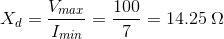[math] rac{F_1}{F_2} = rac{P_1}{P_2} = rac{50}{60} ewline \Rightarrow 6P_1 = 5P_2 [/math] For maximum speed, no. of poles should be minimum. If P1 = 10, P2 = 10 as P1, P2 should be even.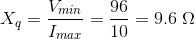[math] N_S = rac{50 imes 120}{10} = 600 \; rpm [/math]

08․ A part an alternator winding consist of eight coils in series, each having an emf of 20 V rms induced in it. Coils are placed in successive slots and between each slot and the next, there is an electrical phase displacement of 30°. Find the emf of eight coils in series
56.91 V.
66.91 V.
76.91 V.
86.91 V.

β = 30° m = 8.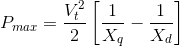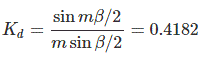Arithmatic sum of voltage induced in coils = 8 X 20 = 160 V Vector sum = Kd X airmetic sum = 0.4182 X 160 = 66.91 V.

09․ A 3-φ alternator has negligible stator resistance. A short circuit test is conducted on this alternator. At a particular speed, a field current of If1 is required to drive the rated armature current. If the speed of the alternator is reduced to half, the field current required to maintain rated armature current
Would be equal to If1/2.
Would be equal to If1.
Woud be equal to 2If1.
Cannot be predicted due to insufficient data.

If speed is reduced to half, then induced emf will be reduced to half or in other words to maintain rated open circuit voltage, the field current required is two times the previous value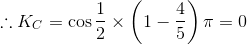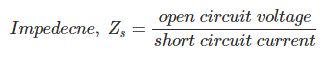But impedence varies with field current and so unless we know that the value of Zs for various field currents it is impossible to maintain rated current at short circuited condition with the half reduced speed.

10․ A single phase, 2 KV alternator has an armature resistance of 0.8 ohms and 4.94 ohms respectively. The voltage regulation of the alternator at 100 a load 0.8 loading power factor is
8.9%.
7%.
14%.
0%.

% Voltage regulation = (EO - VFL) / VFL X 100 where EO = no load voltage. VFL= rated or full load voltage.

<<<8283848586>>>